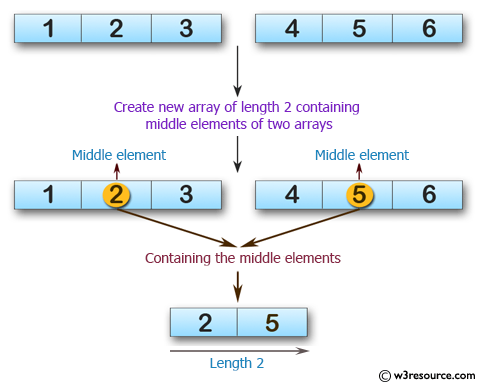﻿ Swift Array Programming Exercise: Create a new array of length 2 containing the middle elements from two give array of integers and length 3 - w3resource# Swift Array Programming Exercises: Create a new array of length 2 containing the middle elements from two give array of integers and length 3

## Swift Array Programming: Exercise-9 with Solution

Write a Swift program to create a new array of length 2 containing the middle elements from two give array of integers and length 3.

Pictorial Presentation:Sample Solution:

Swift Code:

``````func middle_element(_ a: [Int], _ b: [Int]) -> [Int] {
var new_array: [Int] = []
new_array.append(a)
new_array.append(b)

return new_array
}

print(middle_element([1, 2, 3], [4, 5, 6]))
print(middle_element([7, 7, 7], [3, 8, 0]))
print(middle_element([5, 2, 9], [1, 4, 5]))
```
```

Sample Output:

```[2, 5]
[7, 8]
[2, 4]
```

Swift Programming Code Editor:

Improve this sample solution and post your code through Disqus

What is the difficulty level of this exercise?

﻿